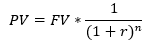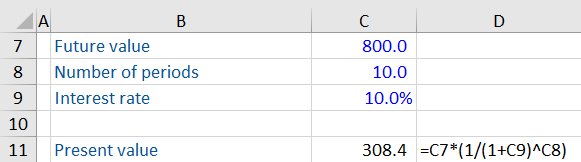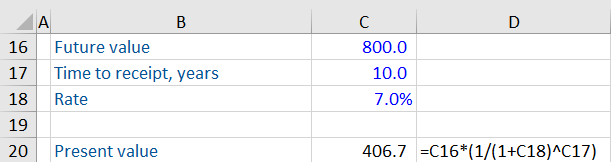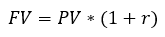## What is Present Value?

Present value is one of the most important concepts in valuation. Simply put, present value involves calculating today’s value of an amount to be received in the future. This helps in comparing different investment alternatives and choosing the most profitable ones.
private investors, or the public via equity capital markets. The term equity financing applies both to publicly listed companies as well as private companies.

## Key Learning Points

• Present value is today’s value of a future income stream based on a defined number of years and discount rate
• The time value of money is the concepts that a known value of cash today is worth more today than receiving the same amount in the future, in other words, investors require an incentive to receive cash at a later date
• Inflation, risk or default and alternative investment opportunities are common reasons why cash is preferred immediately compared to receiving it in the future
• PV can be calculated using the present value formula or the PV function in Excel, =PV( rate, nper, pmt, [fv], type)
• Future value is the value of the cash flow after a pre-defined number of periods and known interest rate

## Time Value of Money

The time value of money (TVM) says that future cash flows are worth less than immediate cash flows. We prefer immediate cash flows for many reasons including:

• Cash now can be spent immediately rather than having to defer spending
• Cash now can be invested to give more cash in the future
• Inflation erodes the buying power of future cash flows
• There is a risk of not receiving the future cash flow due to default

So, all else being equal, a cash flow now would be preferred over the same cash flow in the future. But being able to compare present and future cash flows of different amounts would be useful to help us determine in which situations we would be willing to wait for a future cash flow.

## Present Value Explained

Present value can be calculated using the following formula:Where

PV = Present value

FV = Future value

R = Interest rate

N = Number of periods

The present value (PV) represents today’s value of some future cash flows (denoted by FV in the formula). R or interest rate denotes the level of risk an investor is willing to accept for this investment because future cash flows are not guaranteed, and consequently denotes the return required to compensate for that level of risk. It is possible that the other party may default. Then there are other risks such as economic, political, and technological risks, which may affect future cash flows. Typically, the higher the risk, the increased interest rate. Finally, N represents the number of years or periods needed for this investment.

## Calculating Present Value

Let us understand this concept better with a few examples. You are given an investment proposal that assures a return of 800 after 10 years at an interest rate of 10%. What is the present value of this investment?

Using the formula above, you get the following:

Present value = 800 x 1/ (1+10%)10

= 308.4

Here is the calculation in Excel.You can use the same formula to evaluate different investment alternatives.

## Effect of Discount Rate on Present Value

The discount rate or interest rate can affect the present value of future cash flows. If the discount rate is lower (representing a lower risk and a lower required return), the present value is higher, and vice versa.

Continuing with the same example above, suppose if the interest rate was reduced to 7%, what will be the present value be?The present value is 406.7.

As mentioned above, the discount rate is a function of risk. The riskier an investment, the higher is the discount rate.

Proposal 1 gives a higher 10% return, which implies it is riskier than proposal 2. That means you need to part with a smaller amount today (308.4) to get 800 after 10 years. Whereas proposal 2 involves less risk, and thus offers a lower discount rate. If you want to earn 800 after 10 years from proposal 2, you need to part with a higher amount (406.7) today.

While the present value can be useful in making decisions on investments, as seen above, it is highly dependent on the appropriate discount rate. If the discount rate is not realistic, it can lead to incorrect present values and incorrect investment decisions. In the real world, you cannot be certain of a consistent interest rate over time. Therefore, care should be taken to use the most realistic interest rates or discount rates to get realistic present values.

## Present Value & Inflation

The present value formula can also be used to understand the impact of inflation. We can understand this concept better using the formula for future value:Where

FV = Future value

PV = Present value

R = Interest rate

N = Number of periods

Suppose you have \$100 today. Considering an average inflation rate of 3.24%, what will be the value of the same 100 after 10 years?

Future value = 100 * (1+(-3.24%))10

= 71.9

This calculation can is shown in Excel:Note that inflation is eroding the value of your money, that is why we have used a negative sign in our calculations.